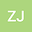•In this paper, we structure a phytoplankton zooplankton interaction system with two delays and Monod-Haldane type functional response, and mainly discuss the affect of $\tau$ and $\tau_1$ to the dynamic behavior of system. Firstly, we give the existence of equilibrium and property of solution. The sufficient conditions ensuring the globally asymptotical stability of the boundary equilibrium are given. The nonexistence of the positive equilibrium ensures the global stability of the boundary equilibrium. Secondly, let $\tau_1=0$ and dynamic behavior of system with one delay ($\tau$) is investigated. The stability switches phenomenon can occur as $\tau$ varying. Then fixed $\tau$ in stable interval, using $\tau_1$ as parameter, it can investigate the effect of $\tau_1$ and find $\tau_1$ can also cause the oscillation of system. Specially, when $\tau=\tau_1$, the system can also occur the stable switching phenomenon, and, under certain conditions, the periodic solution will exist with the wide range as delay away from critical value. Furthermore, using the crossing curve methods, it can obtain the stable changes of positive equilibrium in $(\tau,\tau_1)$ plane. When choosing $\tau$ in the unstable interval, the system still can occur Hopf bifurcation as delays varying. Some numerical simulations are given to indicate the correction of the theoretical analyses. At last, some conclusions are given.